# "Timesheet Calculator" in Microsoft Excel 2010

In this article we will learn about the time calculator which we can use to calculate the total weekly working hours.

To create the Timesheet calculator in Microsoft Excel 2010 we need to use the “TEXT” function, “SUM” and “IF” function.
TEXT function convert a value to text in a specific number format.

Syntax of “TEXT” function:    =TEXT (Value, format_text)

For Example: -

• Cell B2 contains the date 12-Feb-2013
• Write the formula in cell C2 =TEXT(A2,"MMMM") press enter on your keyboard.
• The function will return the month name.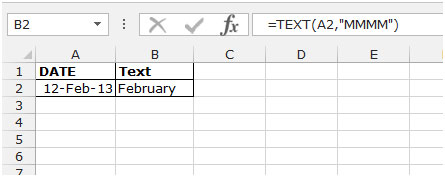SUM function will use to add the hours.

Syntax of “SUM” function:    =SUM (number1, [number2],…..)

For Example: -

• Cell A1 contains the number 240 and A2is 350.
• Write the formula in cell B1=SUM(A1:A2)press enter on your keyboard.
• The function will return the total of cell A1 and A2.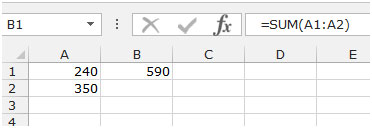IF function will check the logical test according to the putted logics, whethera condition is met and the value if true and another value if false.

Syntax of “IF” function:    =if (logical test, [value_if_true], [value_if_false])

For Example: -

• Cell A1 contains the number 240 and B1 is 350.
• Write the formula in cell C1=IF(A1>B1,"YES","NO")press enter on your keyboard.
• The function will check the logical test about if the cell A1 is greater than B1 then it should be yes otherwise no.
• This function will return No, because cell A1 is not greater than B1.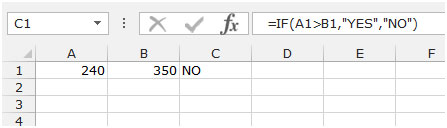Let’s take an example to understand that how we can formulate timesheet calculator in Excel.

We have data to in time and out time date wise. Column A contains the working date, Column B having the in time before the break and Column C out time before the break and column E contains in time after the break and Column F out time after the break. In Cell C10 we are having the total regular hours

In Column G we will calculate the per day working hours. In cell C11 we return the total hours, and in cell C12 we will calculate the overtime hours.To prepare the time sheet calculator follow below mention steps:-

• Select the cell G1 and write the function to return the per day working hours.
• =(C3-B3)+(F3-E3)press enter on your keyboard.
• The function will return the total working hour for a day.
• Copy the formula by pressing the key CTRL+C on your keyboard and paste in the range G4:G8 by pressing the key CTRL+V on your keyboard.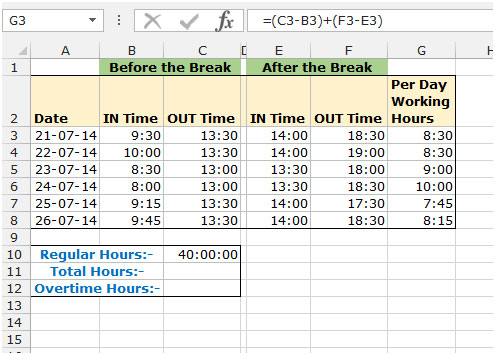To calculate the total hours follow below given steps:-

• Select the cell C11 and write the formula to return the total working hours.
• Just add the per day working hours =SUM(F3:F8).
• Press Enter on your keyboard.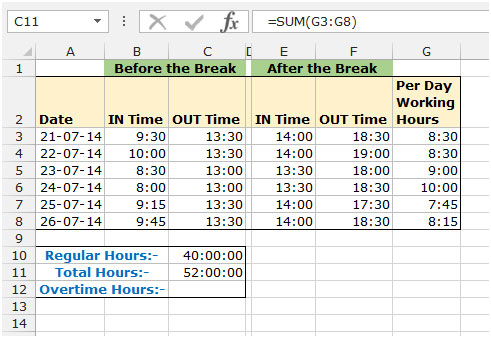To calculate the overtime hours follow below mentioned steps:-

• Select the cell C12 and write the formula to return the overtime hours.
• =IF(C10<C11,C11-C10)
• Press Enter on your keyboard.
• The function will return overtime hours.

This is the way by which you can calculate the working hours by using the formulas in Microsoft Excel.

Note: - For the time formatting use only below showed picture otherwise you will get the wrong result.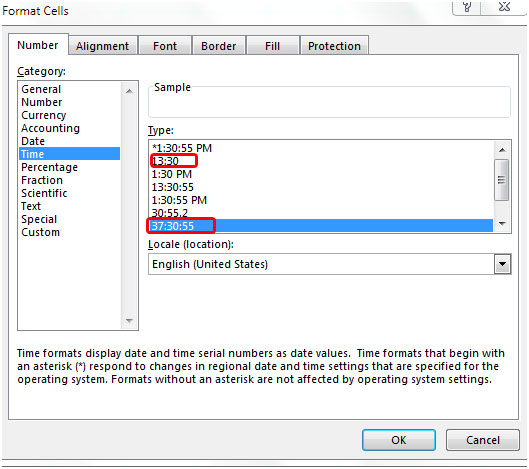Terms and Conditions of use

The applications/code on this site are distributed as is and without warranties or liability. In no event shall the owner of the copyrights, or the authors of the applications/code be liable for any loss of profit, any problems or any damage resulting from the use or evaluation of the applications/code.